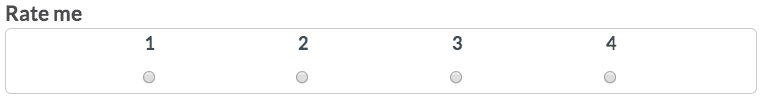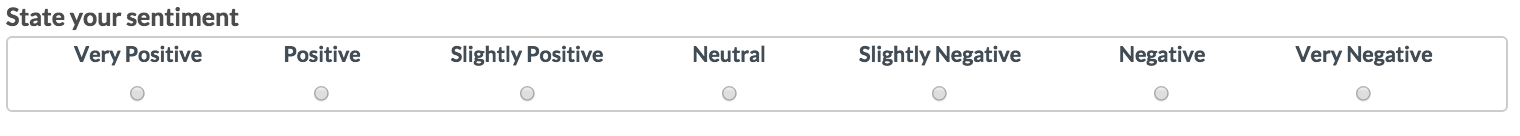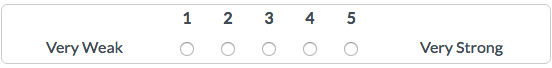Follow

# cml:ratings - Multiple Radios in a Single Line

### cml:ratings

Renders a set of radio buttons in a single line for performing ratings. Accepts all common attributes.

``<cml:ratings label="Rate me" points="4" />``

This type of rating can be used with numerical values or word values. Here we have an example of sentiment related rating with values on each radio:

``````<cml:ratings label="State your sentiment" points="7" >
<cml:rating label="Very Positive"/>
<cml:rating label="Positive"/>
<cml:rating label="Slightly Positive"/>
<cml:rating label="Neutral"/>
<cml:rating label="Slightly Negative"/>
<cml:rating label="Negative"/>
<cml:rating label="Very Negative"/>
</cml:ratings>
``````

cml:ratings can also accept 'from' and 'to' attributes. These will add beginning and ending labels as opposed to addig every label.

``<cml:ratings label="Rate me" points="4" validates="required" from="very weak" to="very strong" />``